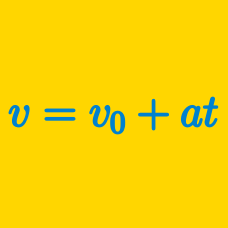Classical Mechanics

# Problem solving with velocity and acceleration - intermediate

The highest rate a public bus may accelerate or decelerate at is $1.4\text{ m/s}^2$ due to safety reasons. If the distance between two stations is $800\text{ m},$ what is the approximate maximum possible instant velocity of the bus while traveling between those two stations?

Assume the road is straight and free of traffic. The bus must come to a complete stop at the stations.

An arrow is uniformly accelerated while traveling $12 \text{ cm}$ until it leaves the bow. If the arrow leaves the bow with a velocity of $6\text{ m/s},$ what is the acceleration of the arrow while it is inside the bow?

The engineer of train $A$ moving with a constant velocity of $36\text{ m/s}$ sights train $B$ on the same track coming towards him at a speed of $22\text{ m/s}.$ The engineer of train $B$ sights train $A$ at the same time. Both drivers immediately apply the brakes, and both trains acquire an acceleration of $-2\text{ m/s}^2$ each. What must be the minimum distance between the two trains to avoid a head-on collision?

A body falling freely from a tower of height $h$ covers a distance of $\frac{4h}{5}$ in the last $6\text{ seconds}$ of its motion before hitting the ground. What is the approximate height of the tower?

The gravitational acceleration is $g=10\text{ m/s}^2,$ and $\sqrt{5}$ is approximately $2.236.$

A body is moving in a straight line with initial velocity $v_0$ and constant acceleration $a.$ The distance traveled by the body at $t=2\text{ s}$ is $18\text{ m},$ and the distance traveled at $t=4\text{ s}$ is $48\text{ m}.$ What is the distance traveled by the body at $t=8\text{ s}?$

×